# GSEB Solutions Class 6 Maths Chapter 6 Integers Ex 6.1

Gujarat Board GSEB Textbook Solutions Class 6 Maths Chapter 6 Integers Ex 6.1 Textbook Questions and Answers.

## Gujarat Board Textbook Solutions Class 6 Maths Chapter 6 Integers Ex 6.1

Question 1.
Write opposites of the following:
(a) Increase in weight
(b) 30 km north
(c) 326 BC
(d) Loss of 700
(e) 100 m above sea level.
Solution:
(a) Decrease in weight
(b) 30 km south
(d) Gain of 700
(e) loo m below sea level

Question 2.
Represent the following numbers as integers with appropriate signs.
(a) An aeroplane is flying at a height two thousand metre above the ground.
(b) A submarine is moving at a depth, eight hundred metre below the sea level.
(c) A deposit of rupees two hundred.
(d) Withdrawal of rupees seven hundred.
Solution:
(a) + 2000 m
(b) – 800 m
(c) + ₹ 200
(d) – ₹ 700Question 3.
Represent the following numbers on a number line:
(a) + 5
(b) – 10
(c) + 8
(d) – 1
(e) – 6
Solution:
(a) Representation of + 5 :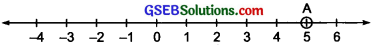In the figure point A represents +5.

(b) Representation of – 10: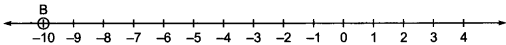In the figure, the point B represents – 10.

(e) Representation of + 8 :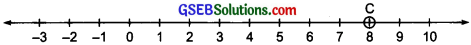In the figure the point C represents + 8.

(d) Representation of – 1:In the figure, the point D represents – 1.

(e) Representation of – 6:In the figure, the point E represents -6.Question 4.
Adjacent figure is a vertical number line, representing integers. Observe it and locate the following points:
(a) If point D is +8, then which point is – 8?
(b) Is point G a negative integer or a positive integer?
(c) Write integers for points B and E.
(d) Which point marked on this number line has the least value?
(e) Arrange all the points in decreasing order of value.
Solution:
(a) The point F represents – 8.
(b) The point G is a negative integer.
(c) The integer at B is + 4. The integer at E is – 10.
(d) The point E corresponds to the least number.
(e) The decreasing order is: D, C, B, A, O, H, G, F, E.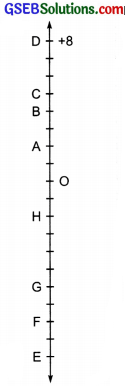Question 5.
Following is the list of temperatures of five places in India on a particular day of the year.(a) Write the temperatures of these places in the form of integers in the blank column.
(b) Following is the number line representing the temperature in degree Celsius.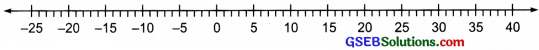Plot the name of the city against its temperature.
(c) Which is the coolest place?
(d) Write the names of the places where temperatures are above 10°C.
Solution: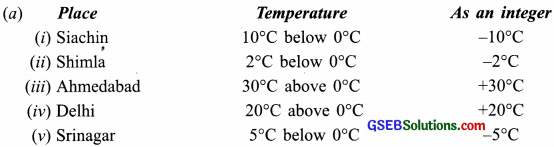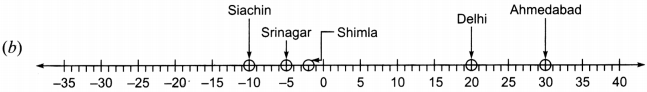(c) Siachin ( – 10°C) is the coolest place.
(d) Delhi (+ 20°C) and Ahmedabad (+ 30°C) are the places where temperatures are above 10°C.Question 6.
In each of the following pairs, which number is to the right of the other on the number line?
(a) 2.9
(b) – 3. – 8
(c) 0, – 1
(d) – 11, 10
(e) – 6, 6
(f) 1, -100
Solution:
The greater integer is on the right of the smaller.
(a) 2,9:
The integer 9 is to the right of integer 2.

(b) – 3, – 8:
The integer – 3 is to the right of integer – 8.

(c) 0, – 1:
The integer O is to the right of integer – 1.

(d) – 11, 10:
The integer 10 is to the right of integer – 11.

(e) – 6, 6:
The integer 6 is to the right of integer – 6.

(f) 1, – 100:
The integer 1 is to the right of integer – 100.Question 7.
Write all the integers between the given pairs (write them in the increasing order).
(a) 0 and – 7
(b) – 4 and 4
(c) – 8 and – 15
(d) – 30 and – 23
Solution:
(a) 0 and – 7: The integers between 0 and
– 7 (in increasing order) are: – 6, – 5, – 4, – 3, – 2 and – 1.

(b) – 4 and 4: The integers between – 4 and 4 (in increasing order) are: – 3, – 2, – 1, 0, 1, 2 and 3.

(c) – 8 and – 15: The integers between – 8 and – 15 (in increasing order) are: – 14, – 13, – 12, – 11, -10 and – 9.

(d) – 30 and – 23: The integers between – 30 and – 23 (in increasing order) are: – 29, – 28, – 27, – 26, – 25, and – 24.

Question 8.
(a) Write four negative integers greater than – 20.
(b) Write four negative integers less than – 10.
Solution:
(a) – 19, – 18, – 17 and – 16 are greater than – 20.
(b) – 11, – 12, – 13 and – 14 are less than – 10.Question 9.
For the following statements, write True (7) or False (F). If the statement is false, correct the statement.
(a) – 8 is to the right of – 10 on a number line.
(b) – 100 is to the right of – 50 on a number line.
(e) Smallest negative integer is – 1.
(d) – 26 is greater than – 25.
Solution:
(a) True .
(b) False; correct statement: – 100 is to the left of – 50 on a number line.
(c) False; correct statement: ‘Greatest negative integer is – 1.
(d) False; correct statement: ‘- 26 is smaller than – 25.’

Question 10.
Draw a number line and answer the following:
(a) Which number will we reach if we move 4 numbers to the right of – 2.
(b) Which number will we reach if we move 5 numbers to the left of 1.
(c) If we are at – 8 on the number line, in which direction should we move to reach – 13?
(d) If we are at – 6 on the number line, in which direction should we move to reach – 1?
Solution:
(a) Starting from – 2 and moving 4 steps towards right (each step being equal to 1 unit) we will reach at 2.
(b) Which number will we reach f we move 5 numbers to the left of 1.
(c) If we are at – 8 on the number line, in which direction should we move to reach – 13?
(d) If we are at – 6 on the number line, in which direction should we move to reach – 1?
Solution:
(a) Starting from – 2 and moving 4 steps towards right (each step being equal to I unit) we will reach at 2.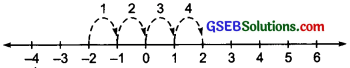(b) Starting from 1 and moving 5 steps, each of 1 unit, towards left, we will reach at number -4.
(c) – 13 < -8 To reach – 13 from – 8, on the number line, we have to move towards the left of – 8. (d) – 1 > – 6
To reach – 1 from – 6, on the number line, we have to move towards the right of – 6.

Like whole numbers, the integers can also be added. We obey the following rules

Rule I: Adding integers with like signs: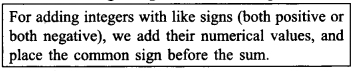Examples:
(i) (+ 2) + (+ 10) (+ 12)
(ii) (- 3) + (- 7) = (- 10)

Rule II: Adding integers of unlike signs: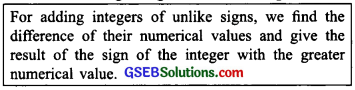(i) (+ 13) + (- 8) = (+ 5)
(ii) (+ 5) + (- 18) = (- 13)